Drawing on top of art pieces to fool a classification network.

Differentiable rasterizer was proposed in . It allows for optimizing for parameters of vector graphics primitives with backpropagation. For example, we can place a set of bezier curves on a canvas and oprimize for their width, color and positions such that it fits some raster image. At each step we rasterize our canvas with curves for a given resolution, then compute $L_2$ difference between two raster images (generated and ground truth) and make a gradient descent step on curves parameters. This was proposed in the original paper as painterly rendering.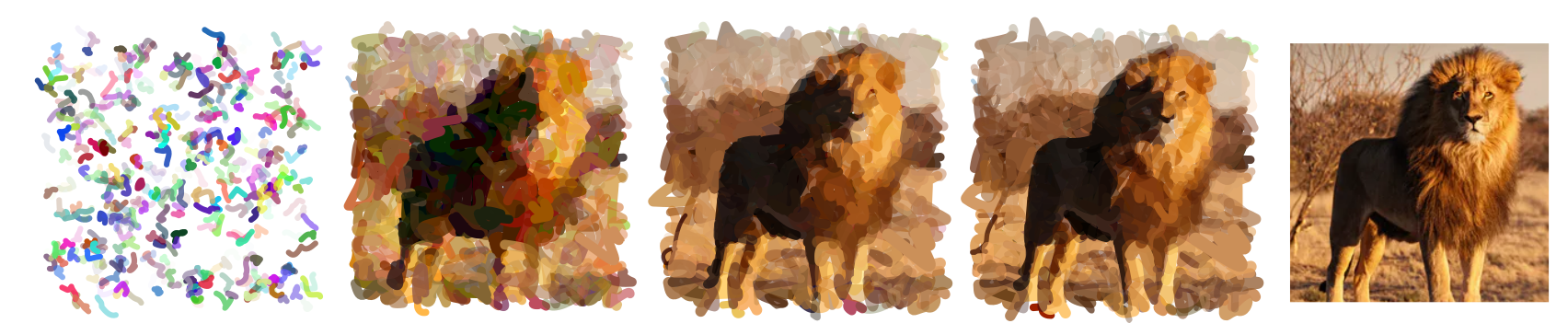Painterly rendering iterations, from left to rigth: random initialization, 50, 100, 200 iterations, target (raster) image.

Disclaimer: This is a project I did for IFT 6756: Game Theory and ML

Can we create adversarial examples using this approach? We fix the number of curves, initialize them at random, and then update for curve parameters using gradient from classification network (pretrained Inception-V3 in our case). The algorithm is similar: rasterize curves, compute classification score with pretrained network, make a gradient step towards maximizing the target class (banana in our case) in curve parameters, repeat. This is known as adversarial example and we use the simples white box attack (since we have direct access to classification system). There are other types of attacks (algorithms of creation adversarial examples), we use the simplest one.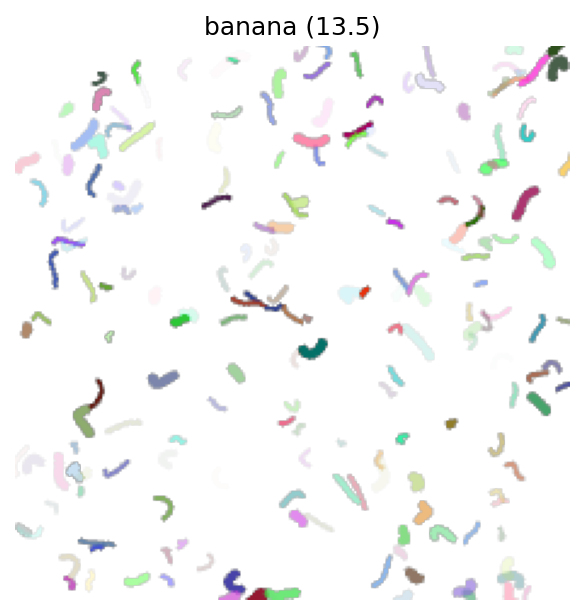Adversarial examples obtained from randomly initialized curves on a canvas. Rasterized image (left) and svg (right).

Now what if we first do some “painterly rendering” steps on randomly initialized images, and then run adversarial examples pipeline? We hope to achieve some meaning in resulting image.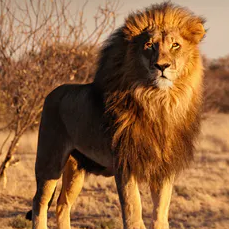Adversarial examples obtained as a combination of painterly rendering (10 iterations towards image on the left), and steps maximizing classification score for targeted class (banana).

Finally, let’s draw curves on top of raster image aiming to create adversarial examples. I call this adversarial vandalism as we destroy the original work by small colored strokes on top.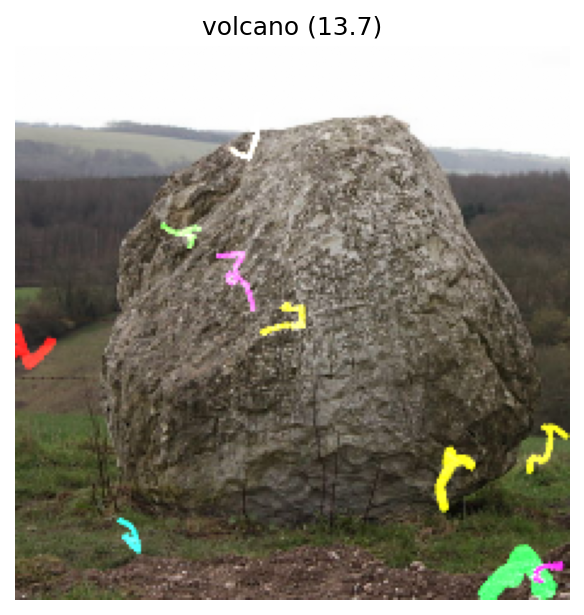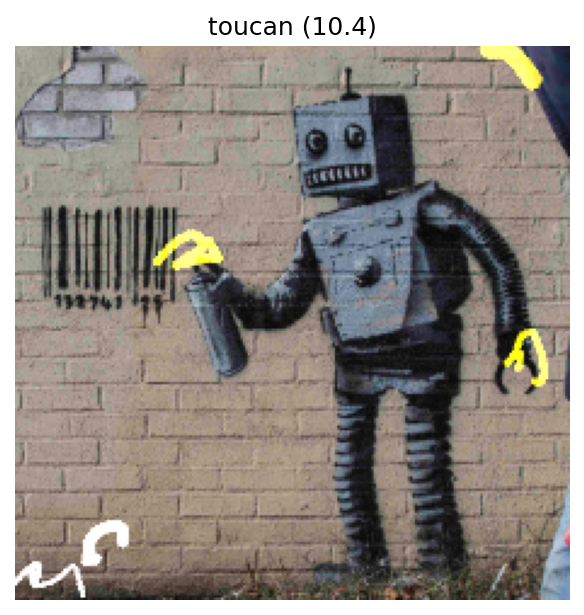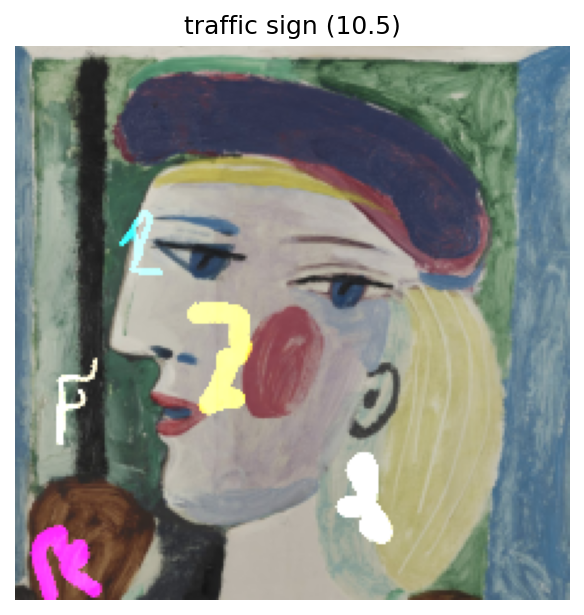Adversarial examples creating by drawing 10 bezier curves on top of a raster image. After placing them at random we optimize curve parameters (positions, width, color) to maximize classification score for a target class.

Upd: A bit of inspiration in my blogpost

-->